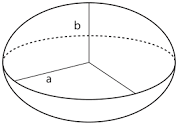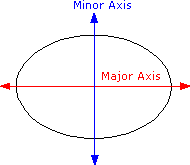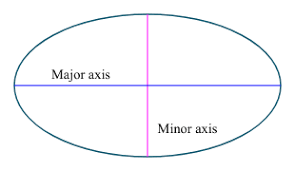## How to Calculate and Solve for the Axis and Volume of an Ellipsoid | The Calculator EncyclopediaThe image above is an ellipsoid.

To compute the volume of an ellipsoid, three essential parameters are needed and this parameters are axis (a)axis (b) and axis (c).

The formula for calculating the volume of an ellipsoid:

V = 4πabc3

Where;

V = Volume of the ellipsoid
a = Axis of the ellipsoid
b = Axis of the ellipsoid
c = Axis of the ellipsoid

Let’s solve an example;
Given that the axis of the ellipsoid (a) is 9 cm and axis of the ellipsoid (b) is 11 cm with the axis (c) of 14 cm. Find the volume of the ellipsoid?

This implies that;
a = Axis of the ellipsoid = 9 cm
b = Axis of the ellipsoid = 11 cm
c = Axis of the ellipsoid = 14 cm

V = 4πabc3
V = 4π(9 x 11 x 14)3
V = 4π(1386)3
V = (12.56)(1386) / 3
V = (17416.98967) / 3
V = 5805.66

Therefore, the volume of the ellipsoid is 5805.66 cm3.

Calculating the Axis (a) of an Ellipsoid using the Volume of the Ellipsoid, Axis (b) of the Ellipsoid and Axis (c) of the Ellipsoid.

a = V3 / 4πbc

Where;
a = Axis of the ellipsoid
V = Volume of the ellipsoid
b = Axis of the ellipsoid
c = Axis of the ellipsoid

Let’s solve an example;
Find the axis (a) of an ellipsoid when the volume of the ellipsoid is 280 cm3 with an axis (b) of 18 cm and axis (c) of 8 cm.

This implies that;
V = Volume of the ellipsoid = 280 cm3
b = Axis of the ellipsoid = 18 cm
c = Axis of the ellipsoid = 8 cm

a = V3 / 4πbc
a = 280 x 3 / (12.566)(18 x 8)
a = 840 / (12.566)(144)
a = 840 / 1809.504
a = 0.46

Therefore, the axis (a) of the ellipsoid is 0.46 cm.

## How to Calculate and Solve for the Perimeter of an Ellipse | Nickzom CalculatorThe image above is an ellipse.

To compute the perimeter of an ellipse, two essential parameters are needed and this parameters are axis (a) and axis (b).

The formula for calculating the perimeter of an ellipse:

P = π [3(a + b) – √((3a + b)(a + 3b))]

Where;

P = Perimeter of the ellipse
a = Axis of the ellipse
b = Axis of the ellipse

Let’s solve an example;
Given that the axis of the ellipse (a) is 19 cm and axis of the ellipse (b) is 31 cm. Find the perimeter of the ellipse?

This implies that;
a = Axis of the ellipse = 19 cm
b = Axis of the ellipse = 31 cm

P = π [3(a + b) – √((3a + b)(a + 3b))]
P = 3.142 [3(19 + 31) – √((3 x 19 + 31)(19 + 3 x 31))]
P = 3.142 [3(50) – √((57+ 31)(19 + 93))]
P = 3.142 [150 – √((88)(112))]
P = 3.142 [150 – √(9856)]
P = 3.142 [150 – 99.28]
P = 3.142 [50.72]
P = 3.142 x 50.72
P = 159.26

Therefore, the perimeter of the ellipse is 159.26 cm.

## How to Calculate and Solve for the Axis and Area of an Ellipse | Nickzom CalculatorThe image above is an ellipse.

To compute the area of an ellipse, two essential parameters are needed and this parameters are axis (a) and axis (b).

A = πab

Where;
A = Area of the ellipse
a = Axis of the ellipse
b = Axis of the ellipse

Let’s solve an example;
Find the area of an ellipse when it has an axis (a) of 10 cm and an axis (b) of 17 cm.

This implies that;
a = Axis of the ellipse = 10 cm
b = Axis of the ellipse = 17 cm

A = πab
A = 3.142 x 10 x 17
A = 534.14

Therefore, the area of the ellipse is 534.14 cm2.

Calculating the Axis (a) of an ellipse using Area of the ellipse and Axis (b) of the ellipse.

a = A / πb

Where;
a = Axis of the ellipse
A = Area of the ellipse
b = Axis of the ellipse

Let’s solve an example;
Find the axis (a) of an ellipse when the area of the ellipse is 210 cm2 with an axis (b) of 19 cm.

This implies that;
A = Area of the ellipse = 210 cm2
b = Axis of the ellipse = 19 cm

a = A / πb
a = 210 / 3.142 x 19
a = 210 / 59.70
a = 3.52

Therefore, the axis (a) of an ellipse is 3.52 cm.